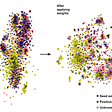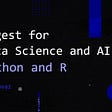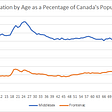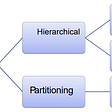# Survival Analysis-A theoretical perspective

Survival Analysis is a branch of machine statistics where the outcome variable is the time it takes until an event occurs. For example, a study in which patients afflicted by a virus or a life-ending disease are tested to determine the survival time depending one the features of the patient eg age, gender, other related diseases etc. Survival analysis is also applied in other domains such as mechanical engineering in assessing system reliability and in marketing to assess how customers churn in a subscription.

However, while it is very similiar to a regression problem there is one primary difference . A lot of the subjects in the study might not experience the event. For example in a customer churn example a lot of the customers might still be customers and using the service. In survival analysis , this is called censored data.

Censoring can come through multiple type:

1. Right Censoring: In this process the event time is as large as the observed time. For example, in a study of customers going through a life threatening disease, some customers will be able to survive through the experiement and in this case the event will not have occurred.
2. Left Censoring: In this type of experiment, the observed time will be as larger than the event time. For example a study of pregnant women where the study time is more than 9 months.
3. Interval Censoring: This is where we do not know the event time but we do not know that it falls in some interval. For example surveying a patient once a month in order to assess whether the event has occurred or not.

The survival function basically returns the probability distribution of an event occurring after time t. Mathematically it can be represented as

S(t)=P(T>t)

where the survival function S(t) gives us the probability function of a subject surviving after time T. A typical survival curve is downward sloping indicating that as time t increases the probability of survival decreases. On a practical level, it is very intuitive as generally as the time passes the probability of a patient from a disease or a customer churning reduces.

One could also argue that one could also compute the probability of the patient dying at time t however we cant do that because of censoring . Censoring does not allow us to compute the probability of an event happening so instead we calculate the probability of survival at a specified time.

Hazard Function

The hazard function is opposed to the survival function. It is the event rate. The hazard at time t is the potential per time for the event to occur.

Mean & Median Survival Time

The mean survival time of a subject can be calculated by the area under the survival curve from zero to survival time. It could be calculated by taking the integral of the survival function with respect to time.

The median survival time is the time at which the survival probability is 0.5.

# Estimating the probability distribution

There are multiple non-parametric & parametric approaches of modelling the distribution of a survival function. .

Weibull distribution

One of the most popular approaches is Weibull distribution which are ideal for modelling the failure of machines. A sample Weibull distribution looks like the below

A Weibull distribution allows us to extend the function in an exponentially by changing its parameter. A b<1 indicates that the hazard rate is expected to fal while a b>1 implies that the hazard rate is expected to increase.

Exponential Distribution

In an exponential distribution the hazard rate is expected to be constant. Mathematically it can be represented as follows:

Hazard Function : h(t)=p

Survival function: S(t)=e^-pt

Density Function: pe^pt

An exponential distribution is ideal where the event rate is constant over time.

# Comparision of models

One of the most common ways to assess the accuracy of a survival model is the Brier score. The Brier score gives the accuracy of any probability metric compared to the actual. Mathematically it can be represented as below:

Brier Score=1/n * Σ(ft — ot)2

where

n=sample size

f=forecasted probability

t=time

o=original probability.

However, the problem with this metric is that it takes a lot of time to train. Another useful metric in assessing could be the AIC(Akaike Information Criterion) mathematically represented as

AIC=-2logL+2k

where L=log-likelihood of the model

and k=number of parameters

# Non-parametric methods

## Kaplan Meier Curve

One of the most common modelling approaches in the Kaplan Meier Estimator.

For survival analysis we assess the variable in this case we will define it as Y as the minimum of the event time or the observed time. In other words

Y=min(E,C)

where E is the event time and C is the censoring time.

Log-Rank test

Suppose we need to see whether there is a difference between the survival distributions of two groups. For example in an experiment we might need to see whether the survival distributions are different for a control or a treatment group. We could also perform a t-test however as explained before we could run into problems due to censoring.

A log-rank test is a large sample chi-square test that uses a chi-square statistic to compare two or more KM curves. The log-rank test can be mathematically represented as below.

W = (X − E(X) )/Var(X).

Other topics

In the next few writeups we will perform Survival analysis on python and will also discuss the Cox’s proportional hazards model. Stay tuned

References

Gareth J., Daniela W., Trevor H. & Robert T. An Introduction to Statistical Learning: with Applications in R (Springer Texts in Statistics). Springer.

--

--

--

## More from Nerd For Tech

NFT is an Educational Media House. Our mission is to bring the invaluable knowledge and experiences of experts from all over the world to the novice. To know more about us, visit https://www.nerdfortech.org/.

## Lookalikes: Finding needles in a haystack## A Step-by-Step Tutorial for Conducting Sentiment Analysis## How to Use These 6 Unusual SQL Functions## Convert Ambiguity into Real Business Needs## Weekly Digest for Data Science and AI: Python and R (Volume 21)## Toronto: No City for Young Kids## How Data drives Business Intelligence?## Applications of Artificial Intelligence in Fire & Safety## Why clustering techniques are relevant in the world of data science?## Hyperparameter tuning for hyperaccurate XGBoost model## The modelling dilemma in Machine Learning — Optimization vs Generalization